# 基于树莓派的智能汽车“智能汽车”这个词可以有几十种不同的含义，这取决于你问的是谁。那么让我们从我对智能购物车的定义开始：

• 触摸屏界面
• 倒车摄像头可让您知道物体是否太近
• 有关汽车的基本信息，例如燃油效率
• 也许蓝牙连接？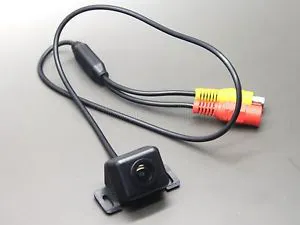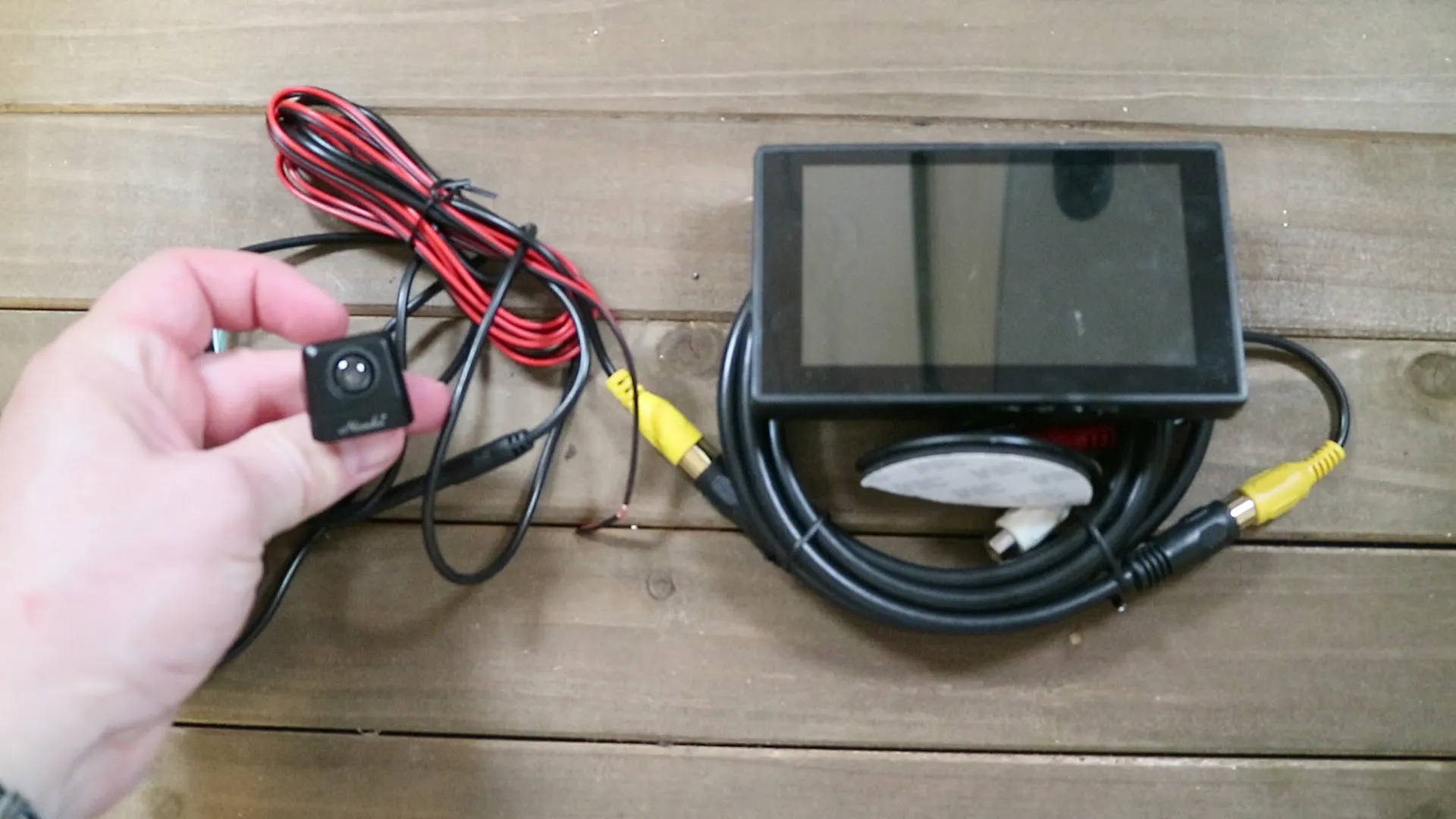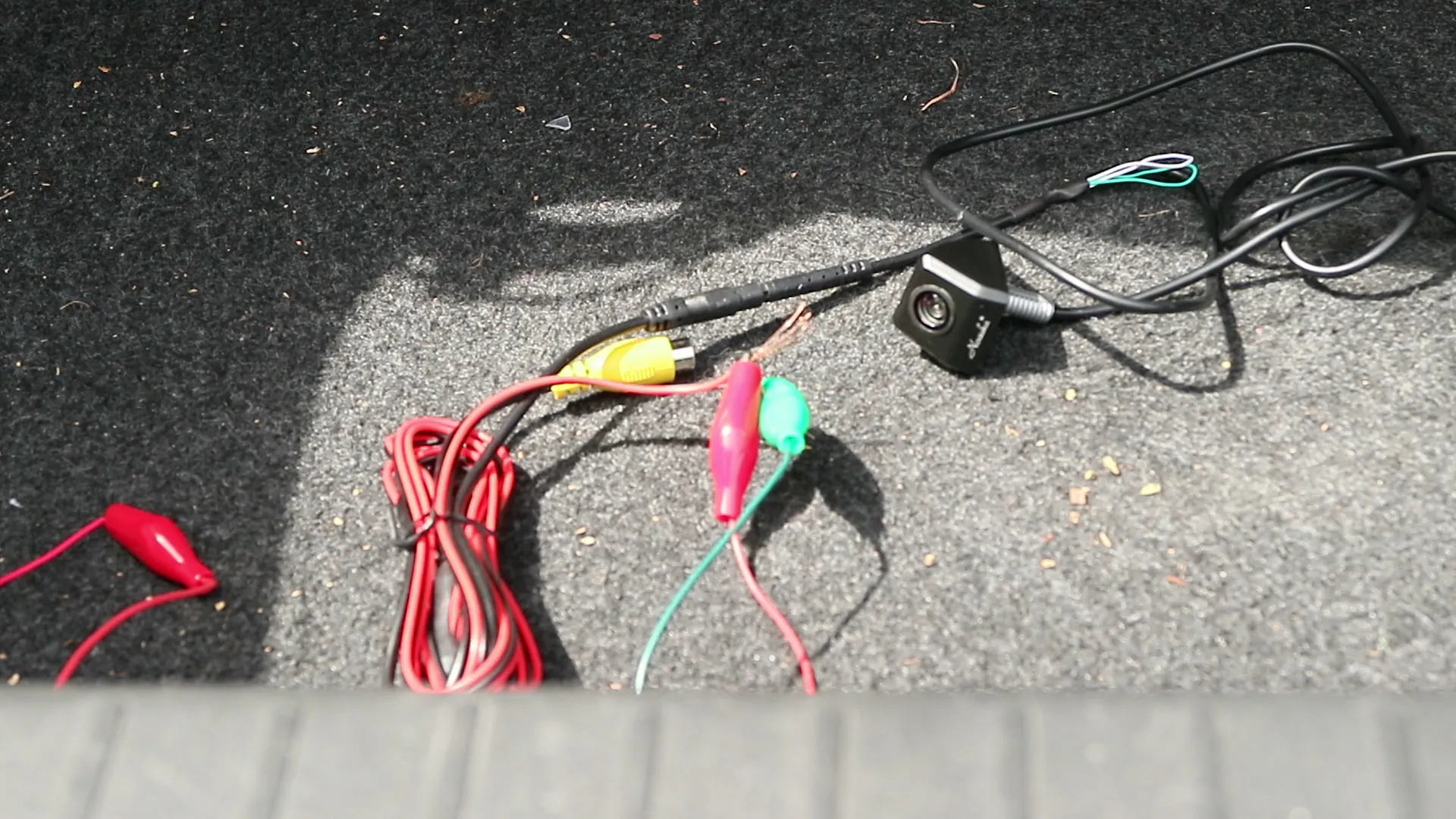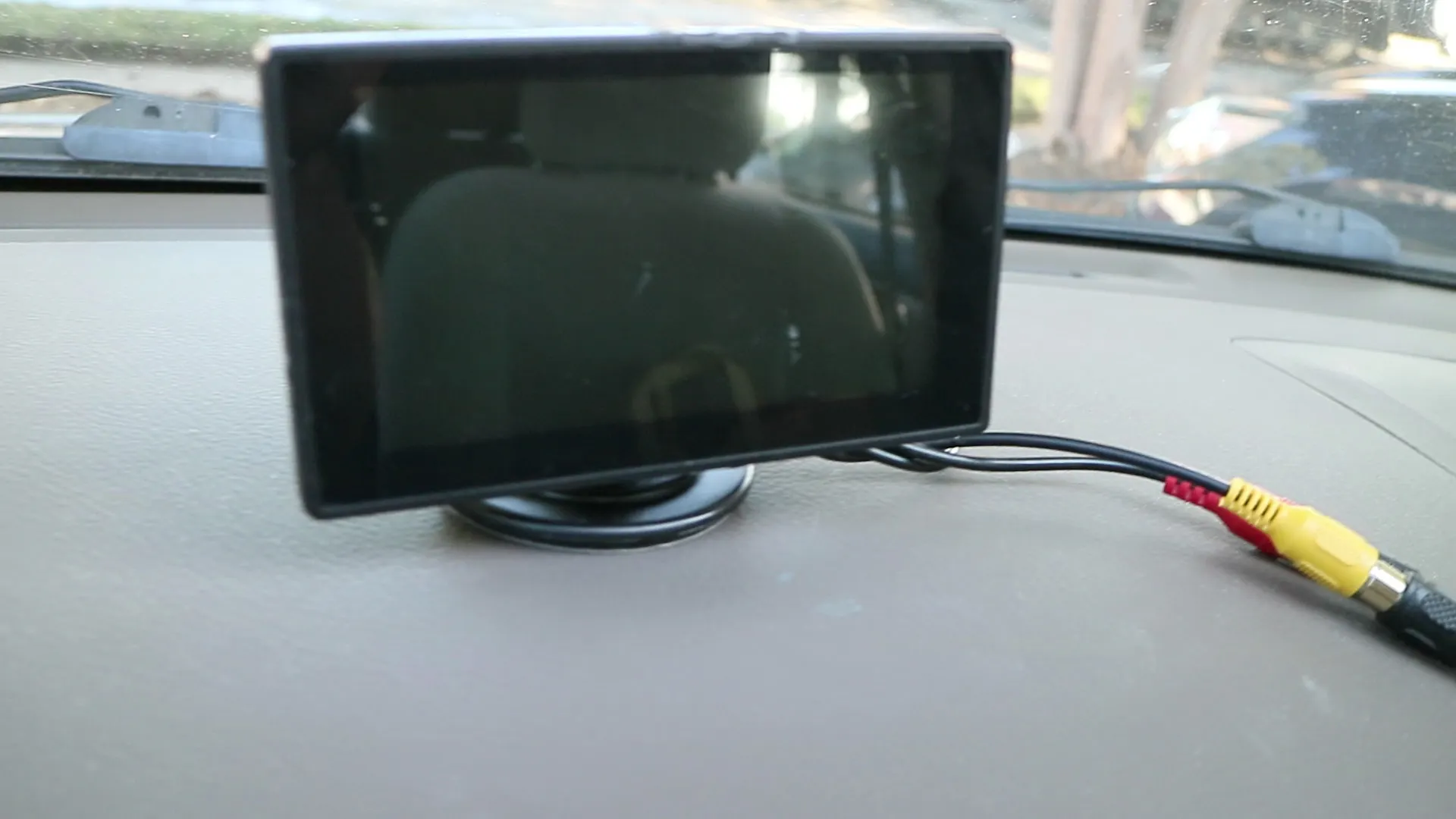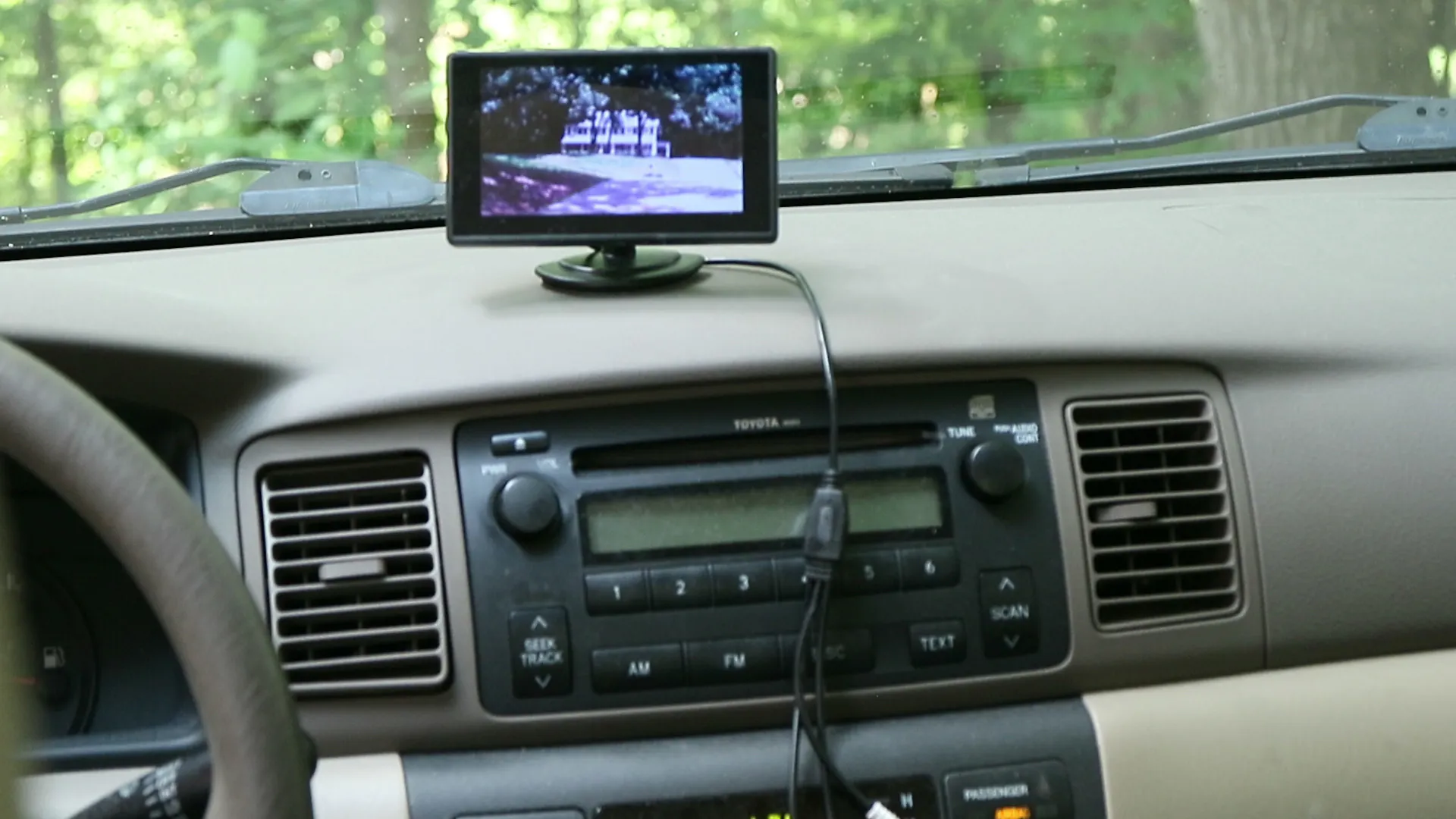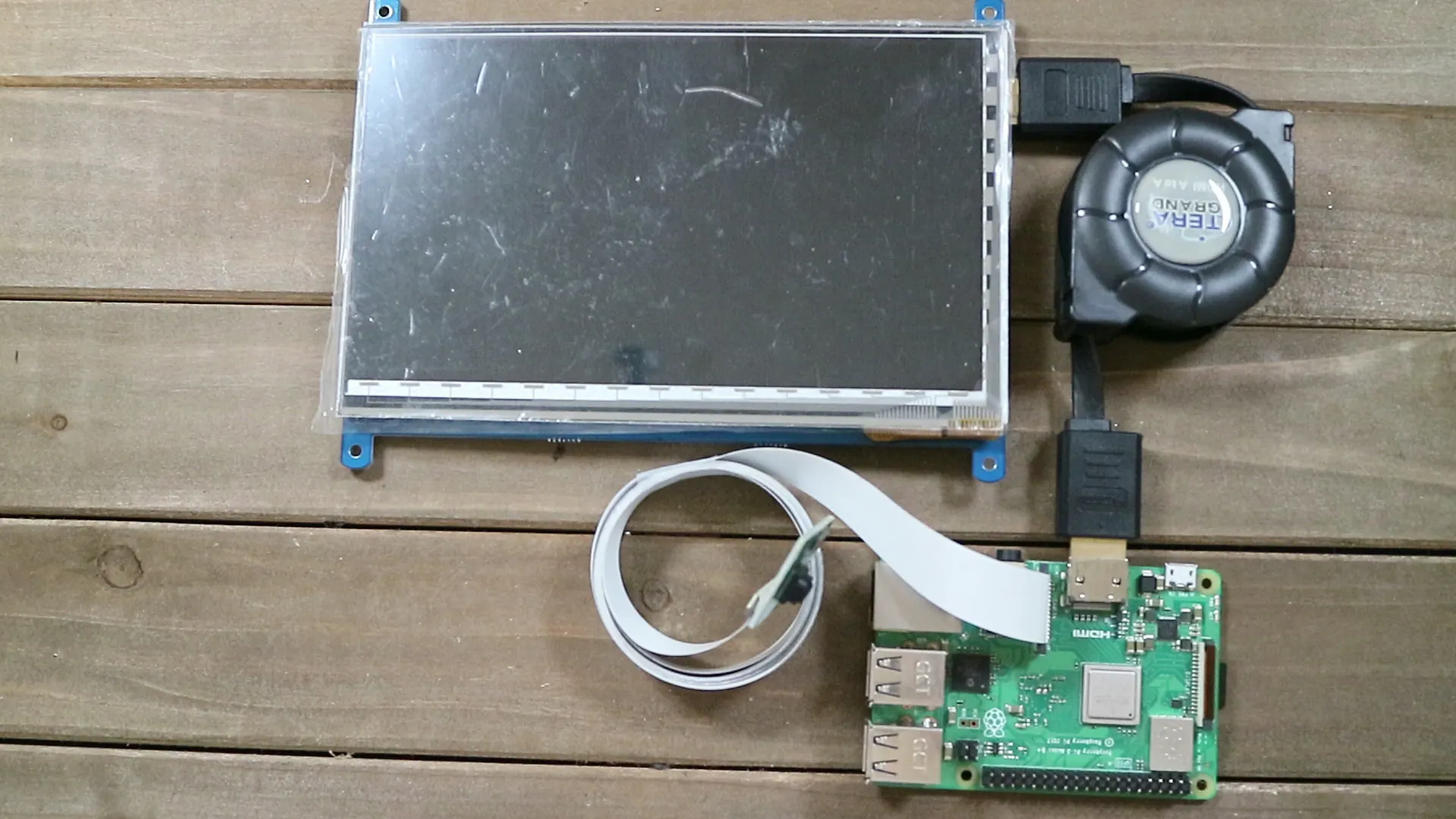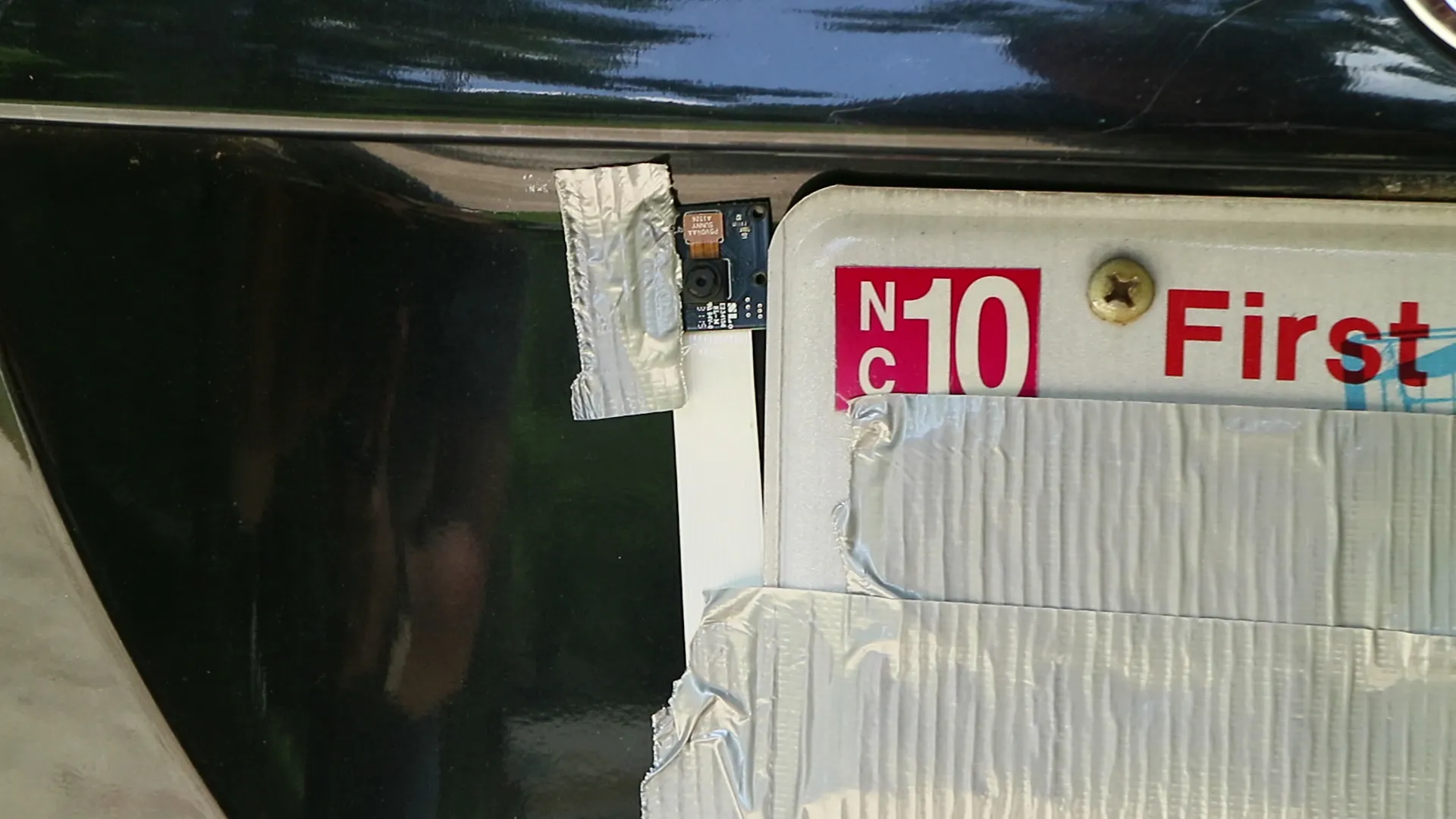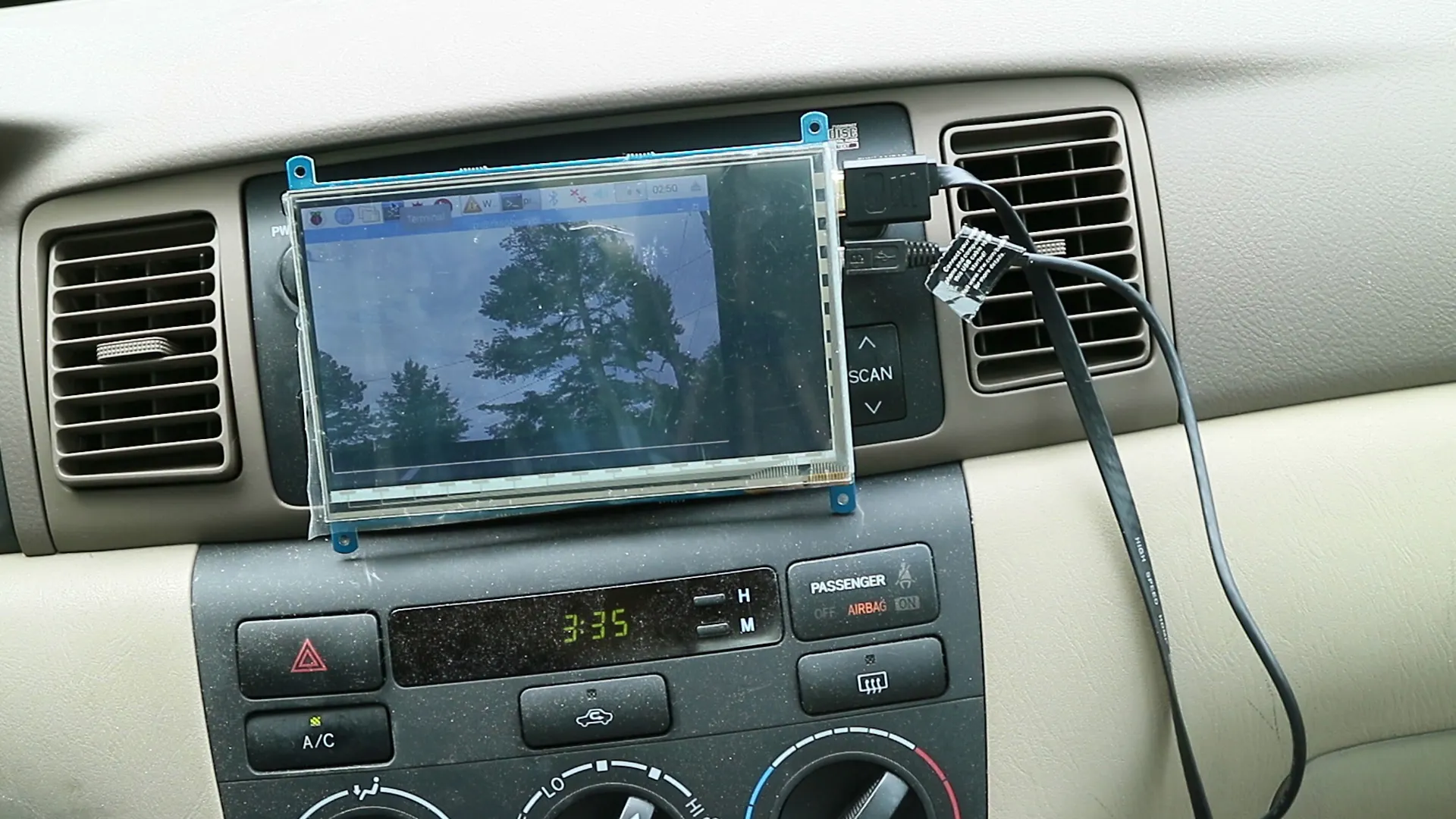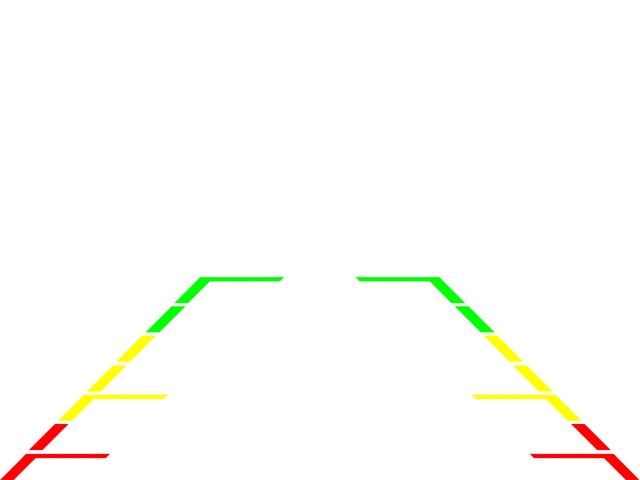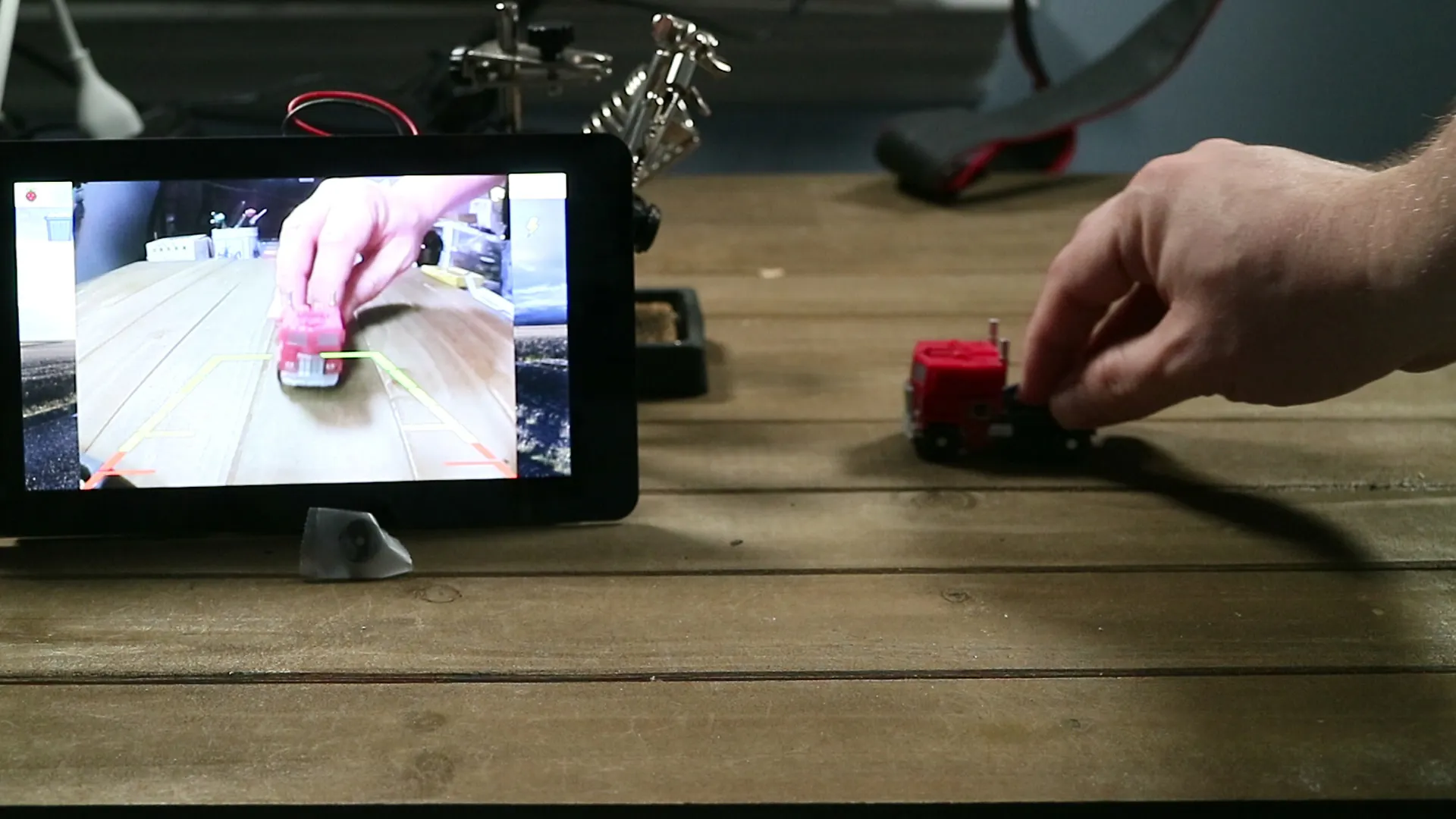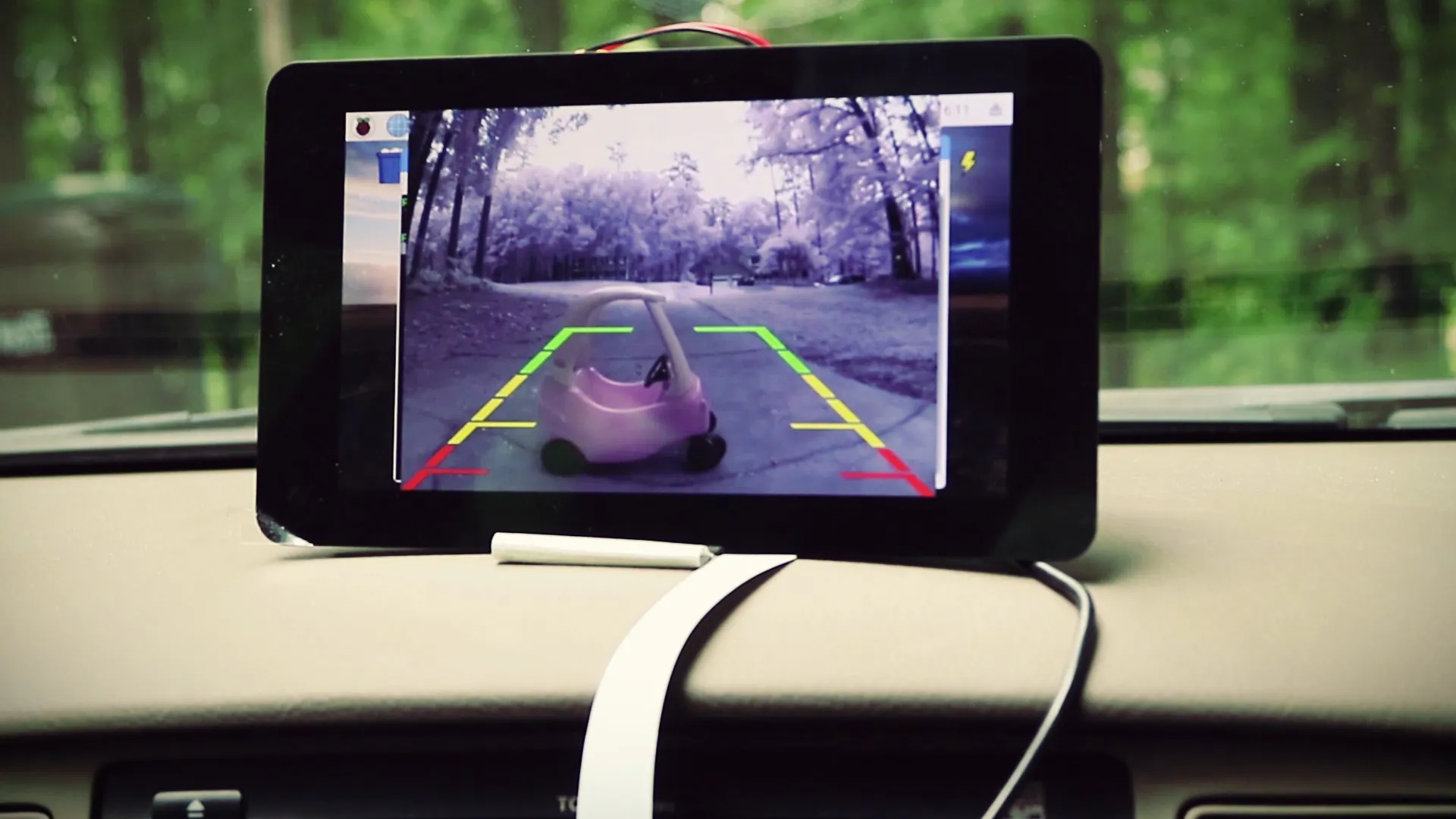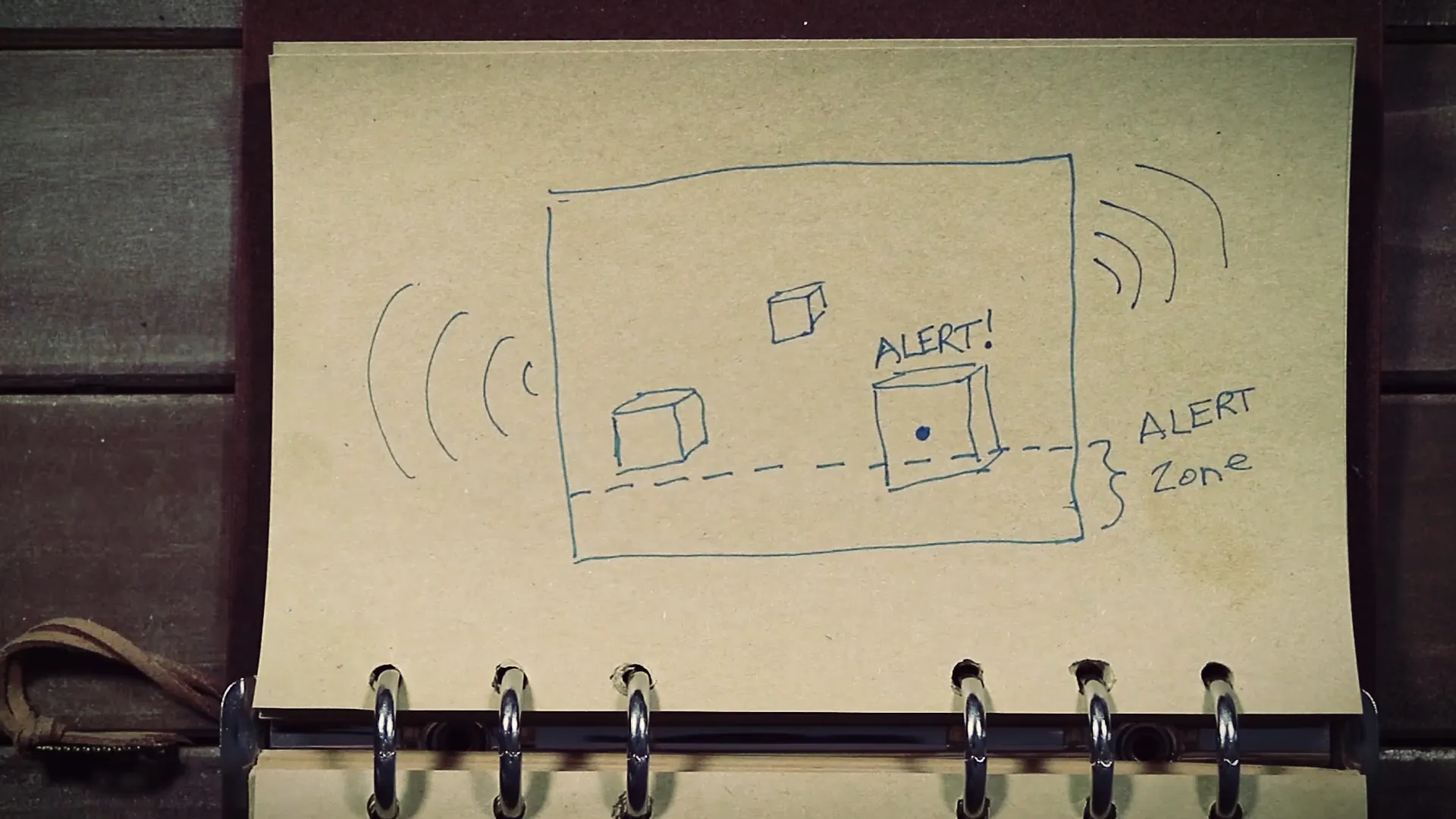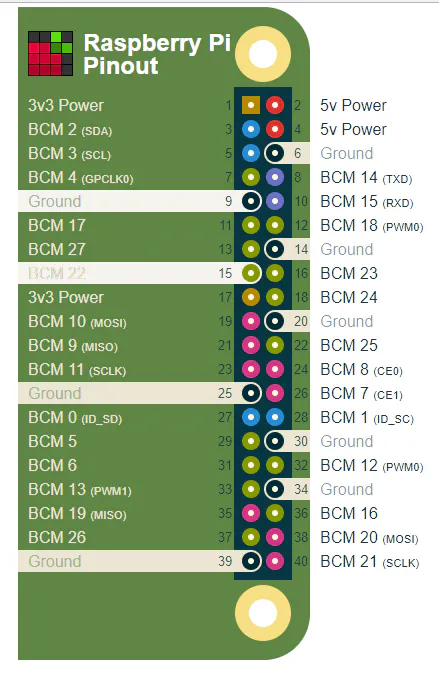import time
import cv2
import numpy as np
from picamera.array import PiRGBArray
from picamera import PiCamera
import RPi.GPIO as GPIO
buzzer = 22
GPIO.setmode(GPIO.BCM)
GPIO.setup(buzzer, GPIO.OUT)
camera = PiCamera()
camera.resolution = (320, 240) #a smaller resolution means faster processing
camera.framerate = 24
rawCapture = PiRGBArray(camera, size=(320, 240))
kernel = np.ones((2,2),np.uint8)
time.sleep(0.1)
for still in camera.capture_continuous(rawCapture, format="bgr", use_video_port=True):
GPIO.output(buzzer, False)

image = still.array
#create a detection area
widthAlert = np.size(image, 1) #get width of image
heightAlert = np.size(image, 0) #get height of image

lower = [1, 0, 20]
upper = [60, 40, 200]
lower = np.array(lower, dtype="uint8")
upper = np.array(upper, dtype="uint8")
#use the color range to create a mask for the image and apply it to the image

dilation = cv2.dilate(mask, kernel, iterations = 3)
closing = cv2.morphologyEx(dilation, cv2.MORPH_CLOSE, kernel)
edge = cv2.Canny(closing, 175, 175)

contours, hierarchy = cv2.findContours(closing, cv2.RETR_TREE, cv2.CHAIN_APPROX_SIMPLE)

threshold_area = 400
centres = []

if len(contours) !=0:

for x in contours:
#find the area of each contour
area = cv2.contourArea(x)
#find the center of each contour
moments = cv2.moments(x)
#weed out the contours that are less than our threshold
if area > threshold_area:

(x,y,w,h) = cv2.boundingRect(x)

centerX = (x+x+w)/2
centerY = (y+y+h)/2

cv2.circle(image,(centerX, centerY), 7, (255, 255, 255), -1)

cv2.putText(image, "ALERT!", (centerX -20, centerY -20), cv2.FONT_HERSHEY_SIMPLEX, 0.5, (255,255,255),2)
GPIO.output(buzzer, True)

cv2.imshow("Display", image)

rawCapture.truncate(0)

key = cv2.waitKey(1) & 0xFF
if key == ord("q"):
GPIO.output(buzzer, False)
break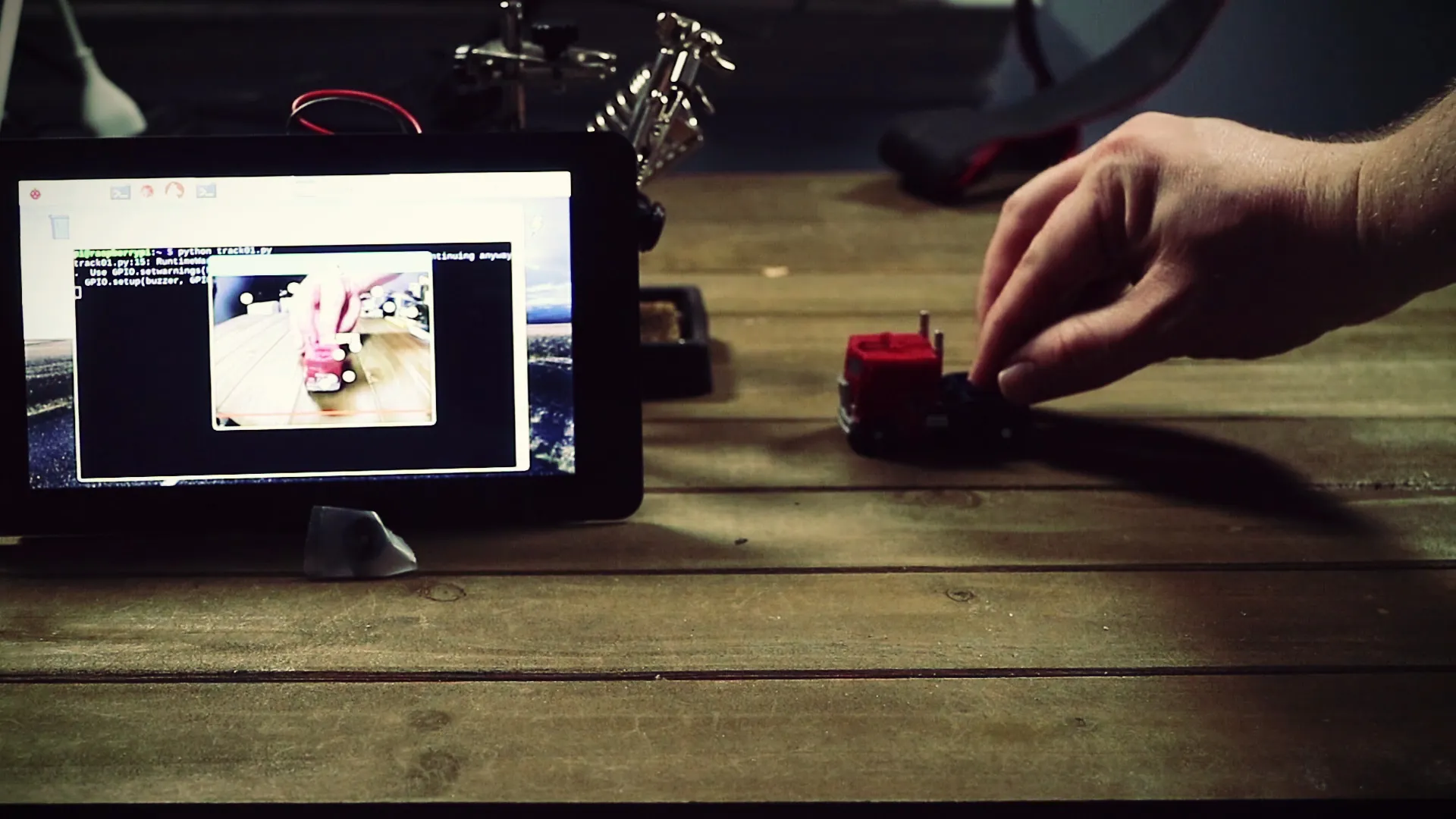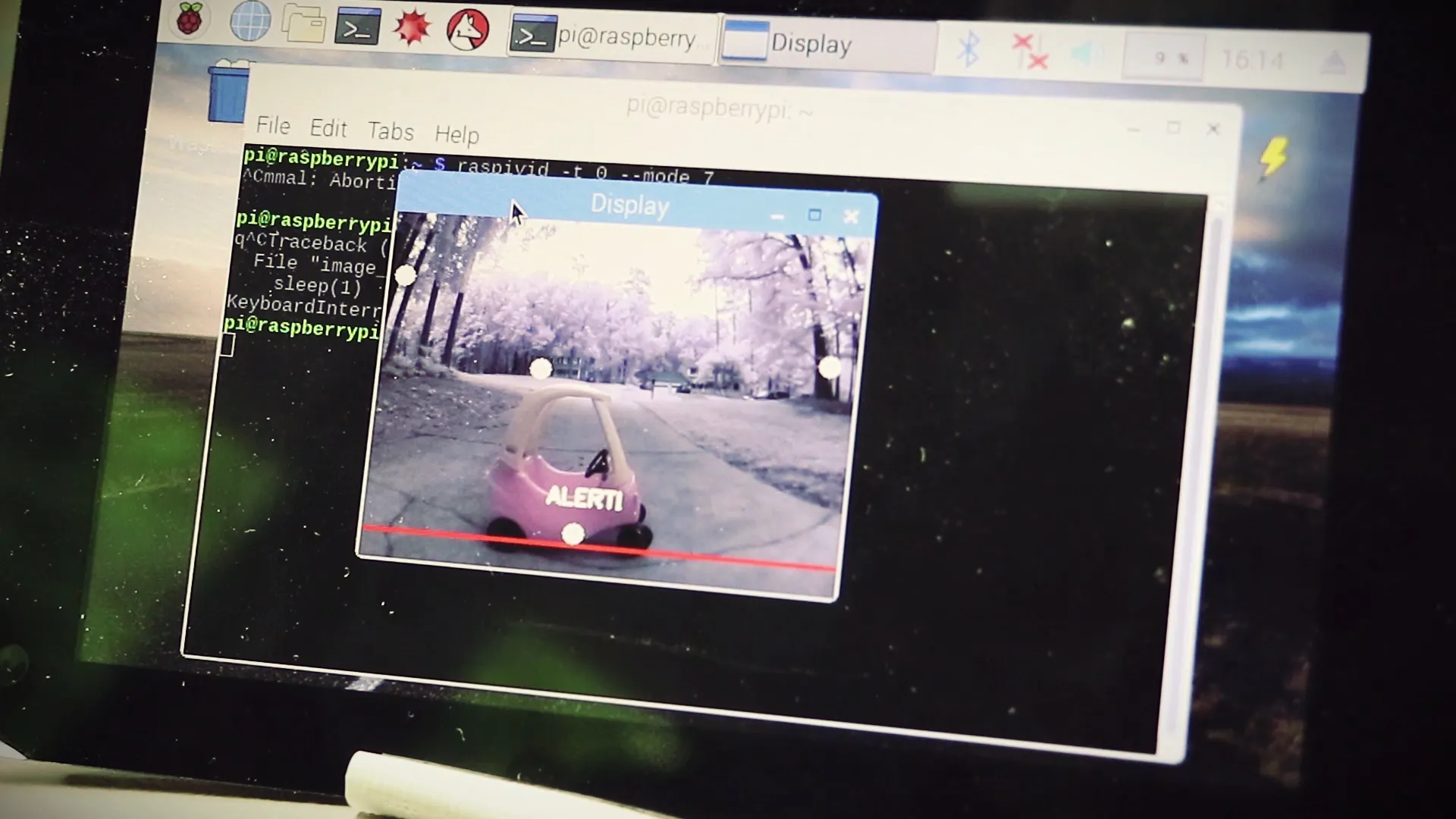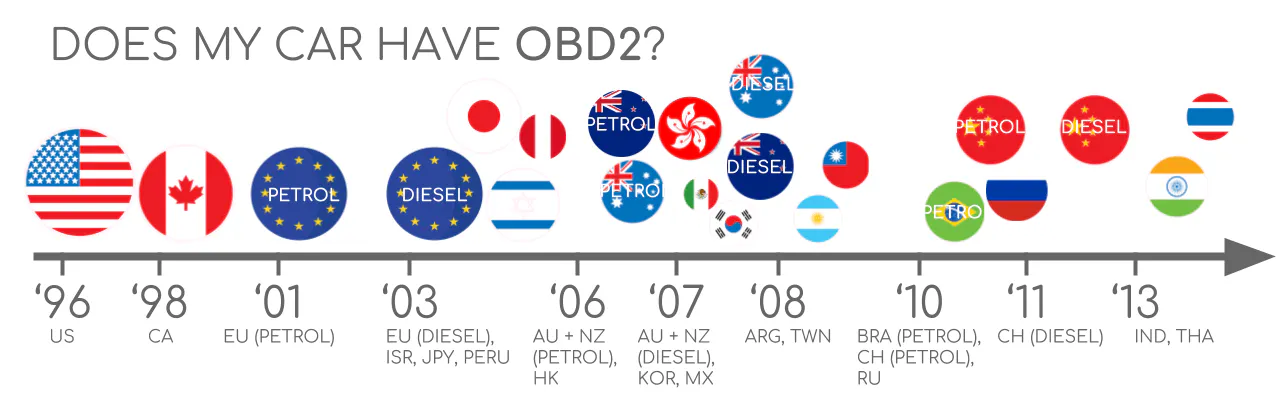OBDII 端口允许您连接到它并读取有关汽车的信息，例如问题、VIN 号码、速度、RPM 等。每当您的“检查引擎”灯亮起时，机械师就会插入它以找出问题所在是并清除问题。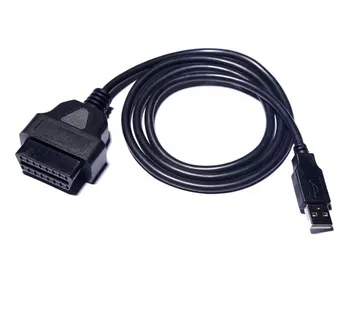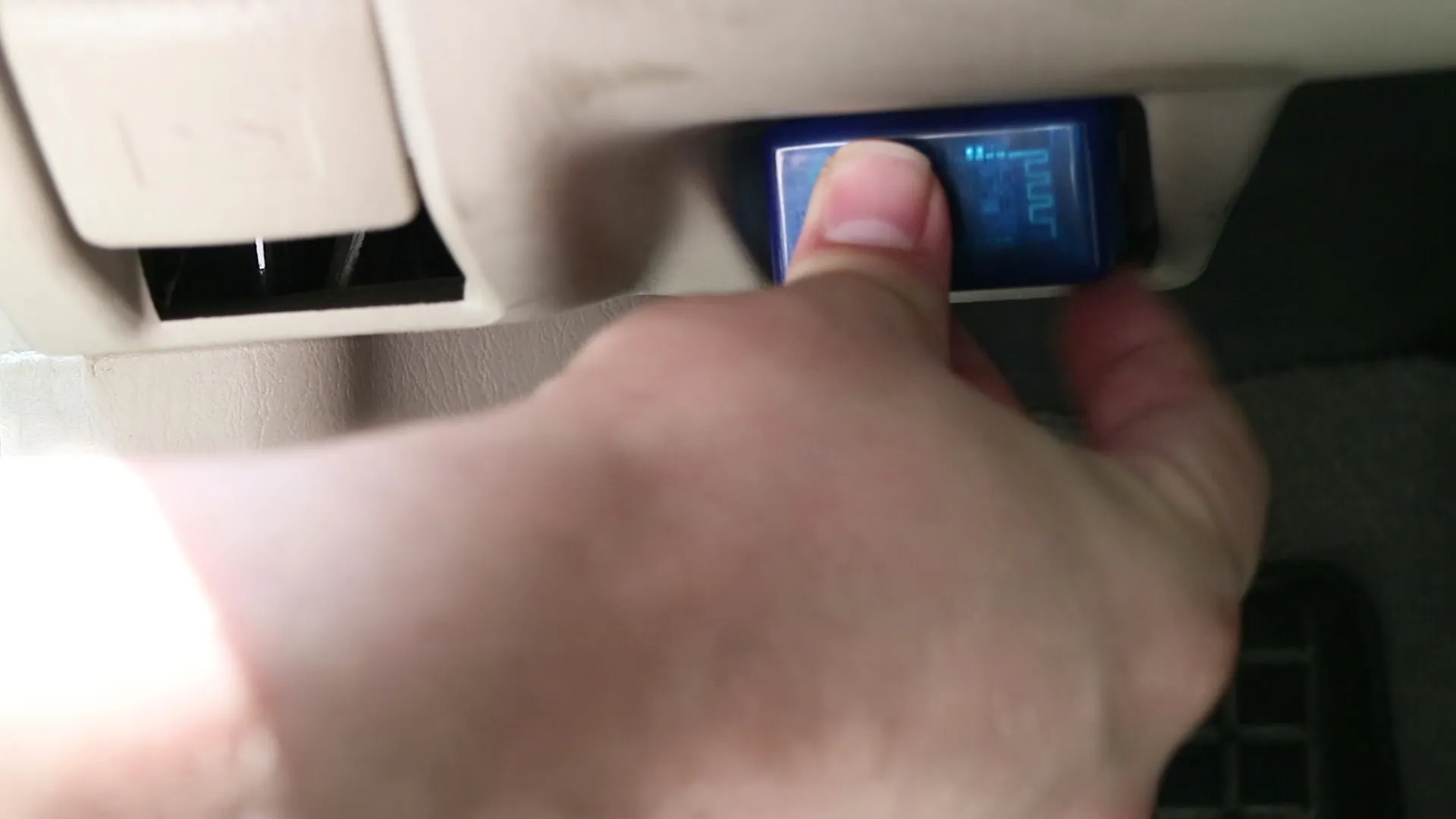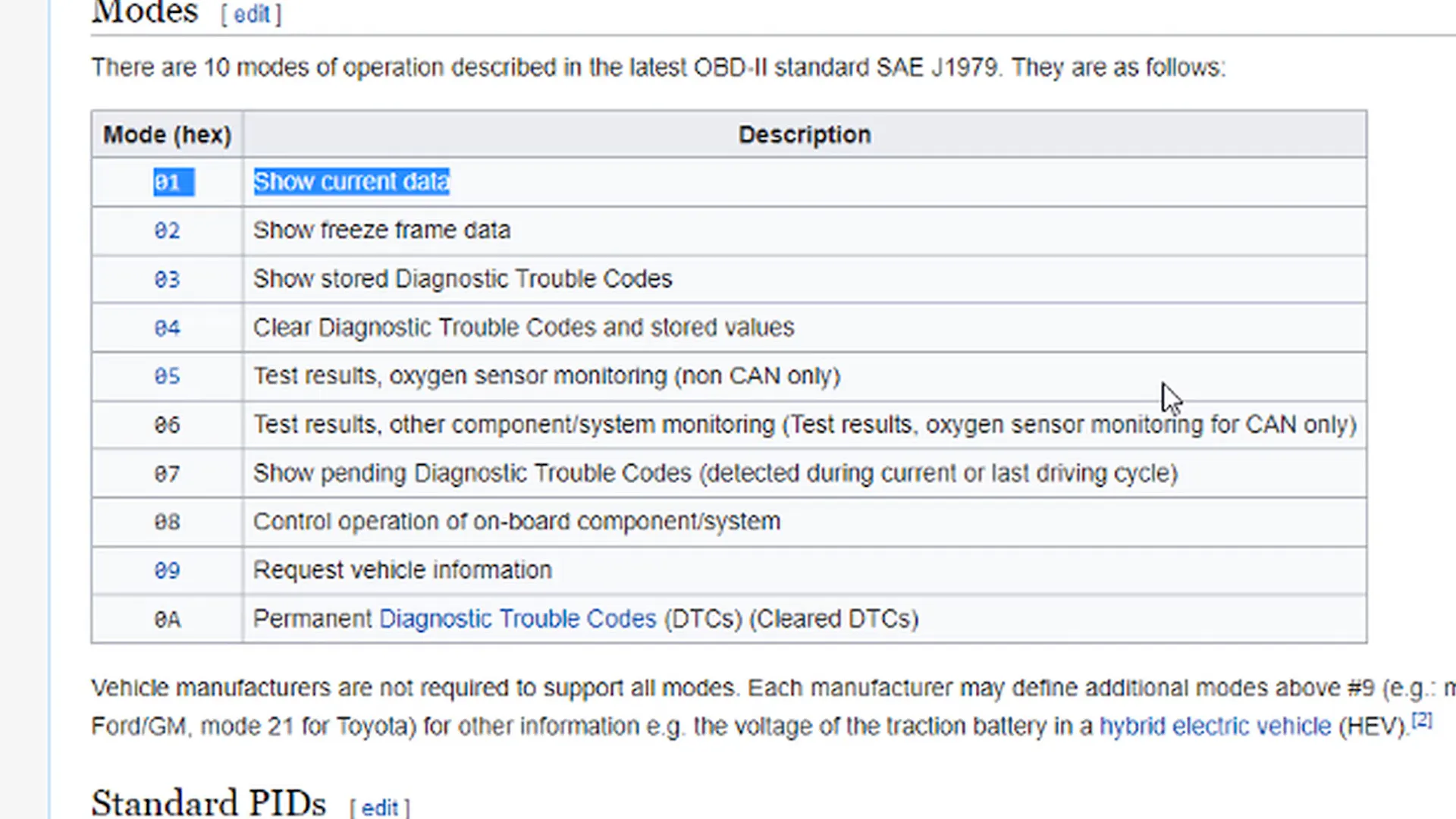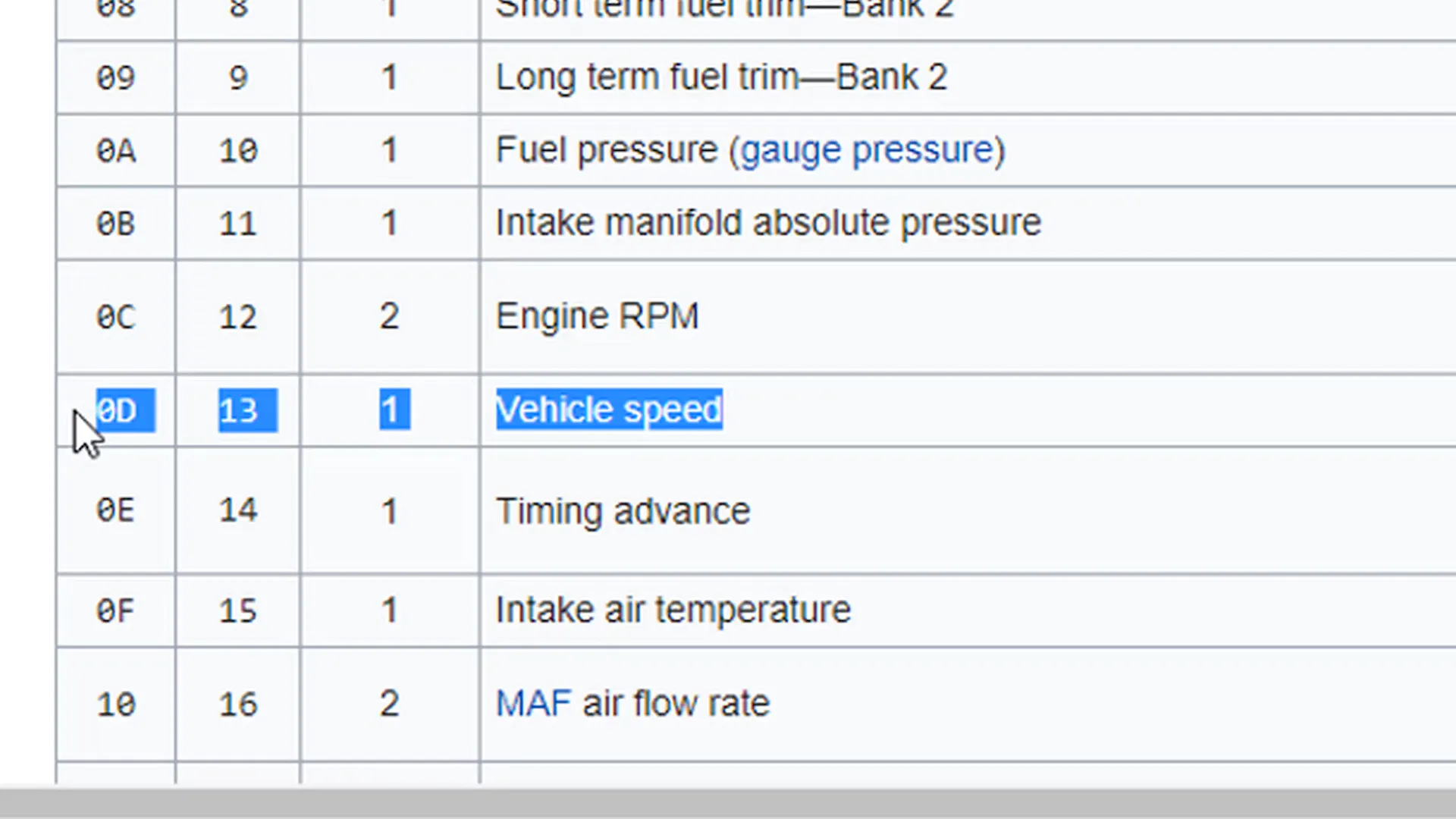• PyQT
• 特金特
• 游戏

Pygame 既快速又简单，因此我将在本教程中使用它。更方便的是，Pygame 预装在树莓派上，所以无需额外安装任何东西！

obd_hud_test.py

import pygame
from pygame.locals import *
import obd
pygame.init()
#connection = obd.OBD()
connect = obd.Async(fast=False)
screen = pygame.display.set_mode((0,0),pygame.FULLSCREEN)
screen_w = screen.get_width()
screen_h = screen.get_height()
circle_y = screen_h/2
circle1_x = screen_w * .25
circle2_x = screen_w * .5
circle3_x = screen_w * .75
speed_text_x = screen_w * .25
speed_text_y = screen_h * .25
rpm_text_x = screen_w * .5
rpm_text_y = screen_h * .25
digitFont = pygame.font.SysFont("Arial", 50)
white = (255,255,255)
black = (0,0,0)
grey = (112, 128, 144)
speed = 0
rpm = 0
def draw_hud():
screen.fill(grey)
pygame.draw.circle(screen, black, (int(circle1_x), int(circle_y)), int(circle_rad), 5)
pygame.draw.circle(screen, black, (int(circle2_x), int(circle_y)), int(circle_rad), 5)
pygame.draw.circle(screen, black, (int(circle3_x), int(circle_y)), int(circle_rad), 5)
speed_text_loc = speed_text.get_rect(center=(speed_text_x, speed_text_y))
rpm_text_loc = rpm_text.get_rect(center=(rpm_text_x, rpm_text_y))
screen.blit(speed_text, speed_text_loc)
screen.blit(rpm_text, rpm_text_loc)
def get_speed(s):
global speed
if not s.is_null():
#speed = int(s.value.magnitude) #for kph
speed = int(s.value.magnitude * .060934) #for mph
def get_rpm(r):
global rpm
if not r.is_null():
rpm = int(r.value.mangitude)
if not l.is_null():
connection.watch(obd.commands.SPEED, callback=get_speed)
connection.watch(obd.commands.RPM, callback=get_rpm)
connection.start()
running = True
while running:
for event in pygame.event.get():
if event.type == KEYDOWN:
if event.key == K_ESCAPE:
connection.stop()
connection.close()
running = False
elif event.type == QUIT:
connection.stop()
connection.close()
running = False
draw_hud()
speedDisplay = digitFont.render(str(speed), 3, white)
rpmDisplay = digitFont.render(str(rpm), 3, white)
screen.blit(rpmDisplay, (circle2_x-(circle2_x/8), circle_y-45))
screen.blit(speedDisplay,(circle1_x-(circle1_x/8), circle_y-45))
pygame.display.update()
pygame.display.flip()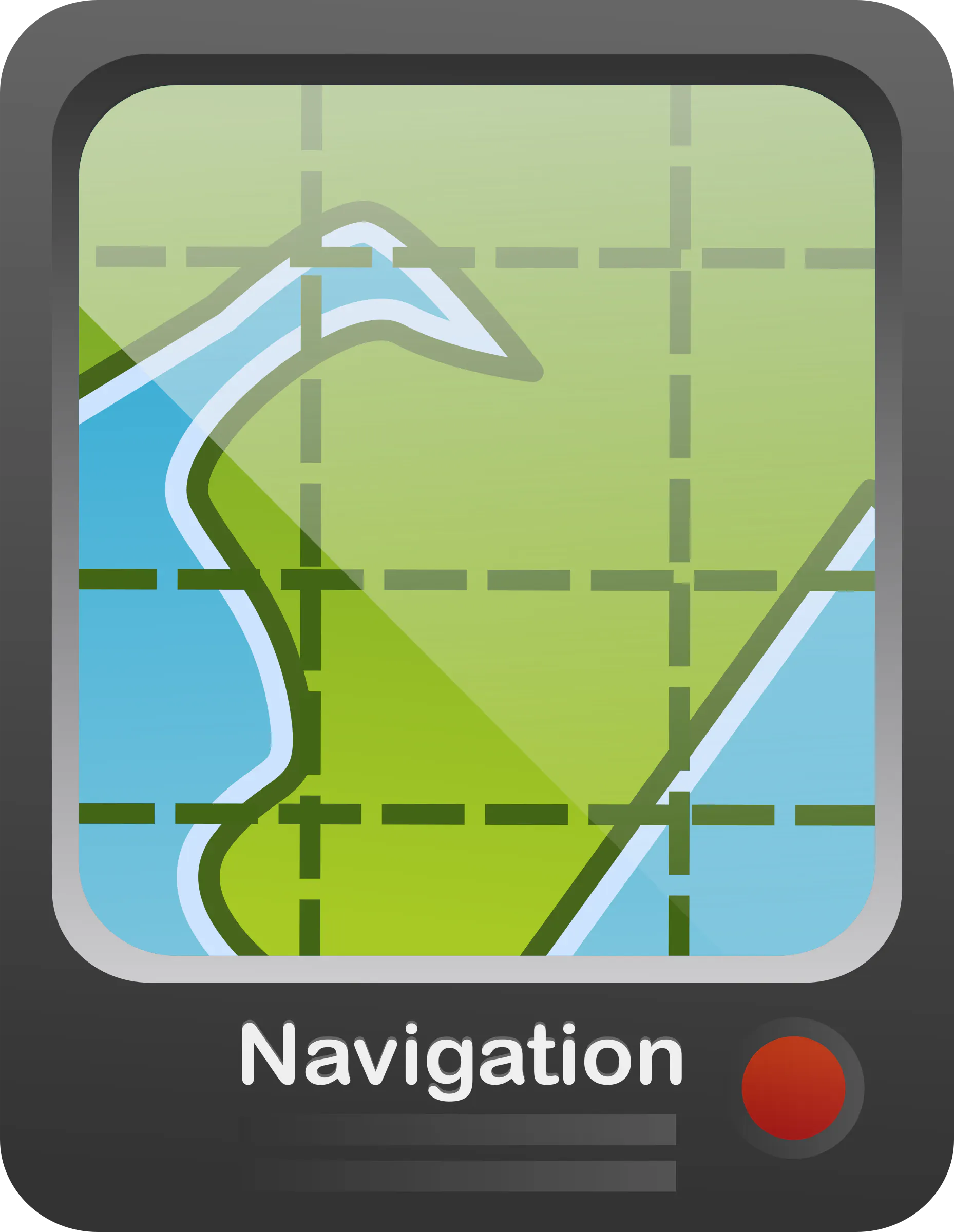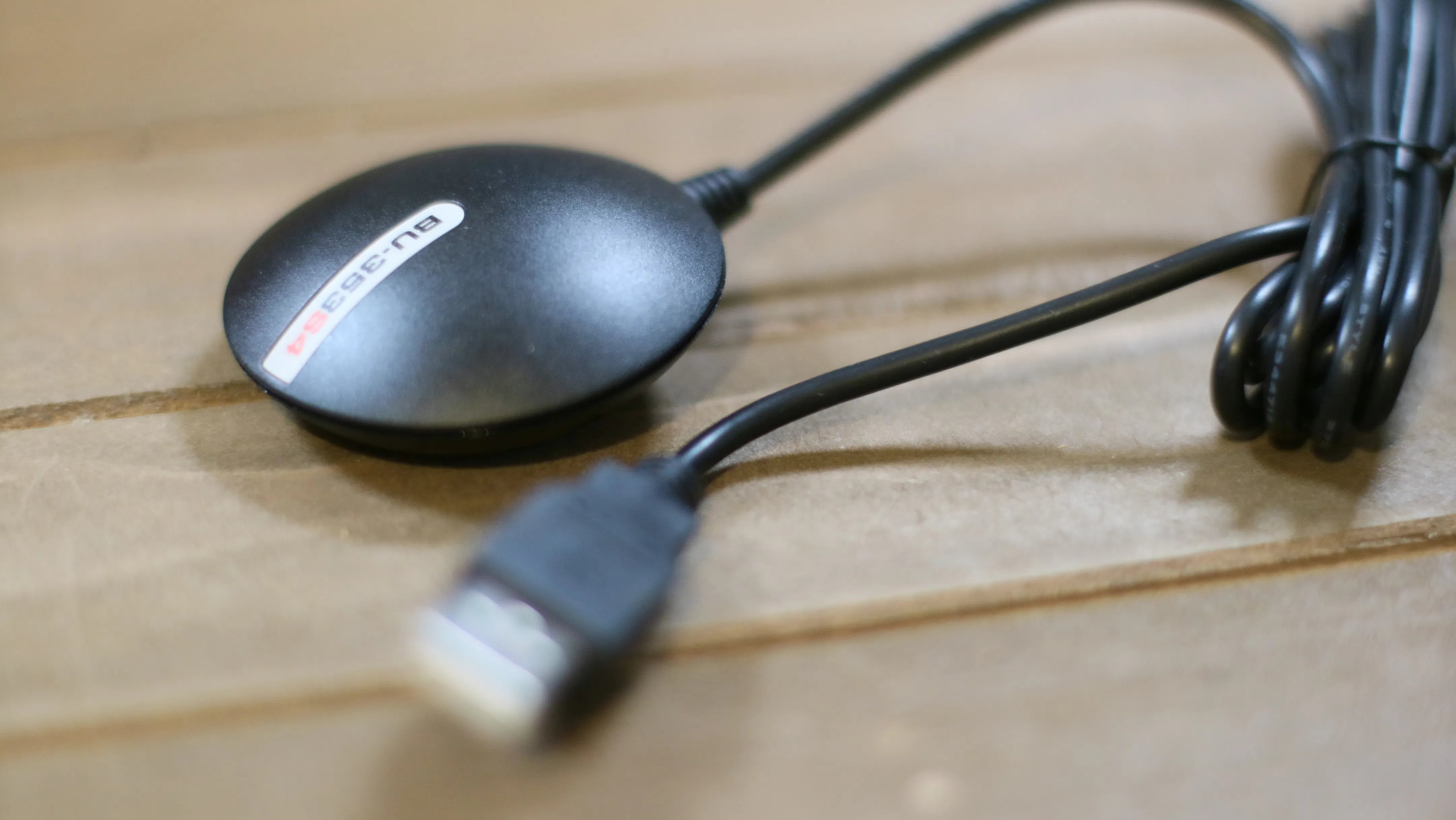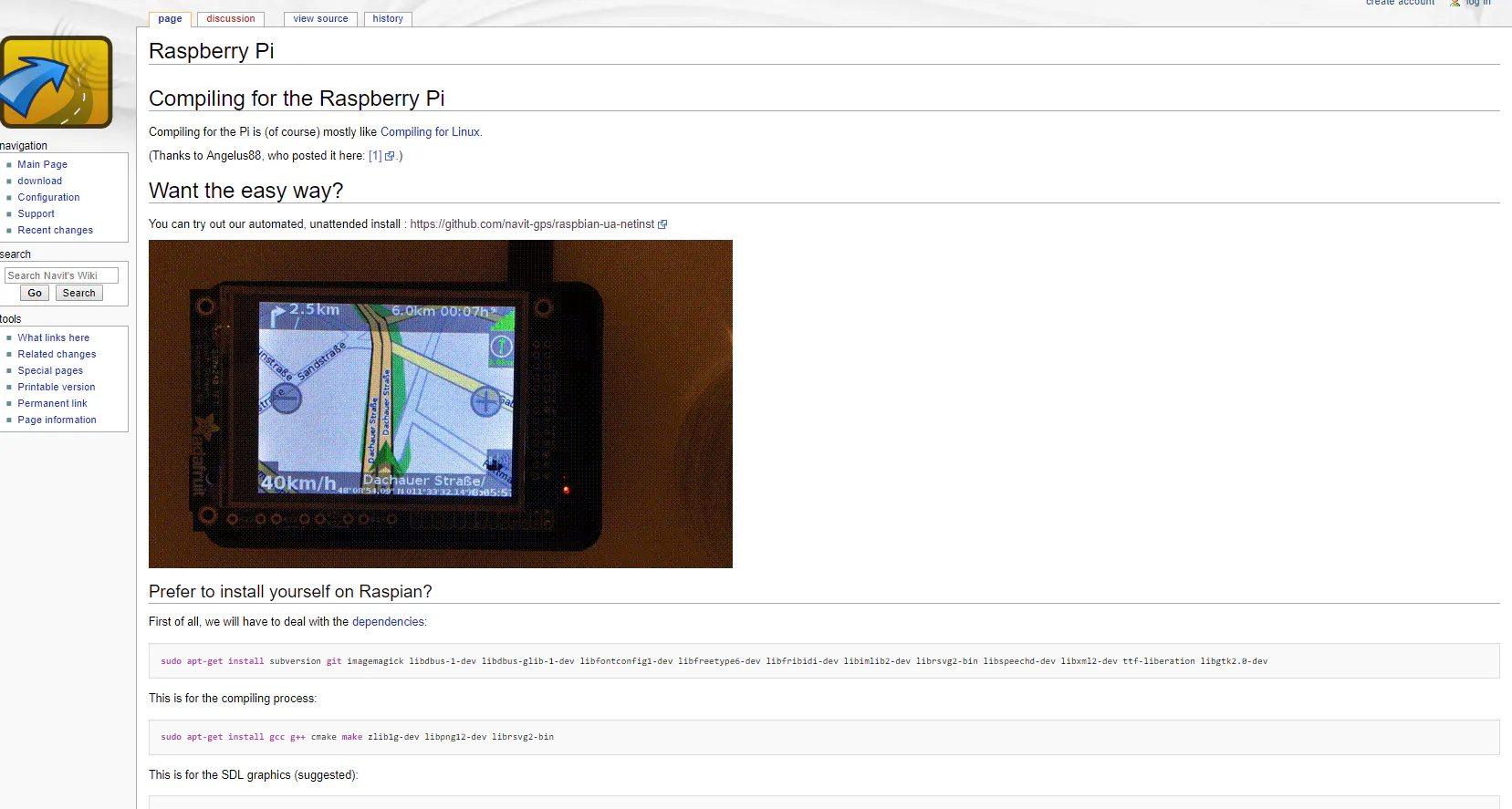加入微信技术交流群关注与非网服务号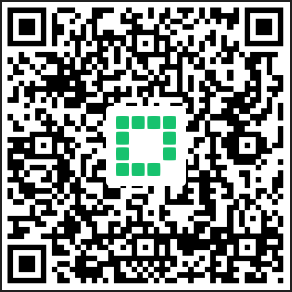加入电路城 QQ 交流群